# Test: Index Numbers- 4

## 40 Questions MCQ Test Business Mathematics and Logical Reasoning & Statistics | Test: Index Numbers- 4

Description
Attempt Test: Index Numbers- 4 | 40 questions in 40 minutes | Mock test for CA Foundation preparation | Free important questions MCQ to study Business Mathematics and Logical Reasoning & Statistics for CA Foundation Exam | Download free PDF with solutions
QUESTION: 1

### The prices of a commodity in the year 1975 and 1980 were 25 and 30 respectively taking 1980 as base year the price relative is :

Solution:

Taking 1980 as base year......

Pn/p0*100

Then,

25/30*100

=83.3333

QUESTION: 2

Solution:
QUESTION: 3

### Cost of living index (C.L.I.) numbers are also used to find real wages by the process of

Solution:
QUESTION: 4

From the following data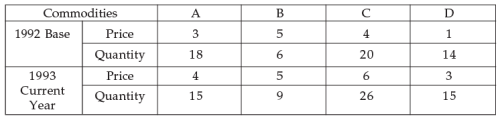Q. The Passche price Index number is :

Solution:
QUESTION: 5

From the following data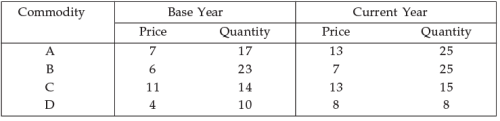Q. The Marshall Edge worth Index number is :

Solution:
QUESTION: 6

The circular Test is an extension of

Solution:
QUESTION: 7

Circular test, an index constructed for the year ‘x’ on the base year ‘y’ and for the year ‘y’ on the base year ‘z’ should yield the same result as an index constructed for ‘x’ on base year ‘z’ i.e. I0,1 x I1,2 x I2,0 equal is :

Solution:
QUESTION: 8

In 1976 the average price of a commodity was 20% more than that in 1975 but 20% less than that in 1974 and more over it was 50% more than that in 1977. The price relatives using 1975 as base year (1975 price relative = 100) then the reduce date is :

Solution:
QUESTION: 9

Time Reversal Test is represented by symbolically is :

Solution:
QUESTION: 10

The prices of a commodity in the years 1975 and 1980 were 25 and 30 respectively, taking 1975 as base year the price relative is :

Solution:
QUESTION: 11

From the following data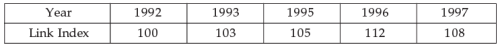Q. (Base 1992 = 100) for the year 1993–97. The construction of chain index is :

Solution:
QUESTION: 12

During a certain period the cost of living index number goes up from 110 to 200 and the salary of a worker is also raised from Rs. 325 to Rs. 500. The worker does not get really gain. Then the real wages decreased by :

Solution:

Firstly, we will calculate by how much the living index number increase, i.e.

200/110 = 1.818

Now, we'll multiply this factor by the wages before increase, so as to calculate the final increased wages for maintaining the right balance.

Rs 325 * 1.818 = Rs. 590.90

Hence, the real wages decrease by Rs.590.90 - Rs.500 = Rs.90.90

QUESTION: 13

Net monthly salary of an employee was Rs. 3000 in 1980. The consumer price index number in 1985 is 250 with 1980 as base year. If the has to be rightly compensated. Then 7th dearness allowances to be paid to the employee is :

Solution:
QUESTION: 14

Net Monthly income of an employee was Rs. 800 in 1980. The consumer price Index number was 160 in 1980. It is rises to 200 in 1984. If he has to be rightly compensated. The additional dearness allowance to be paid to the employee is :

Solution:
QUESTION: 15

When the cost of Tobacco was increased by 50%, a certain hardened smoker, who maintained his formal scale of consumption, said that the rise had increased his cost of living by 5%. Before the change in price, the percentage of his cost of living was due to buying Tobacco is

Solution:
QUESTION: 16

If the price index for the year, say 1960 be 110.3 and the price index for the year, say 1950 be 98.4. Then the purchasing power of money (Rupees) of 1950 will be of 1960 is

Solution:
QUESTION: 17

When index number is calculated for several variables, it is called:

Solution:

⇒  When index number is calculated for several variables, it is called : Composite Index.
⇒  A composite index number is formed by combining two or more index numbers, and a weighting is usually given to each of the indexes in order to calculate the weighted average index number.
⇒  A composite index number is a number that measures an average relative change in a group of relative variables with respect to a base.

QUESTION: 18

The consumer price Index for April 1985 was 125. The food price index was 120 and other items index was 135. The percentage of the total weight of the index is

Solution:
QUESTION: 19

The total value of retained imports into India in 1960 was Rs. 71.5 million per month. The corresponding total for 1967 was Rs. 87.6 million per month. The index of volume of retained imports in 1967 composed with 1960 (= 100) was 62.0. The price index for retained inputs for 1967 our 1960 as base is

Solution:
QUESTION: 20

During the certain period the C.L.I. gives up from 110 to 200 and the Salary of a worker is also raised from 330 to 500, then the real terms is

Solution:
QUESTION: 21

From the following data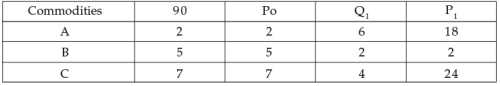Q. Then the fisher’s quantity index number is

Solution:
QUESTION: 22

From the following data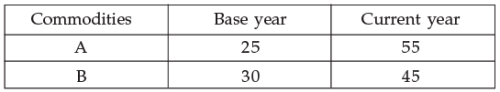Q. Then index numbers from G. M. Method is :

Solution:
QUESTION: 23

Using the following data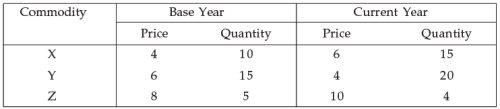Q. The Paasche’s formula for index is :

Solution:
QUESTION: 24

Group index number is represented by

Solution:
QUESTION: 25

The quantity Index number using Fisher formula satisfies:

Solution:
QUESTION: 26

For constructing consumer price Index is used:

Solution:
QUESTION: 27

If the 1970 index with base 1965 is 200 and 1965 index with base 1960 is 150, the index 1970 on base 1960 will be:

Solution:
QUESTION: 28

With regard to Laspeyre and Paasche price index numbers, it is maintained that If the Prices of all the goods change in the same ratio, the two indices will be equal for them the weighting system is irrelevant; or if the quantities of all the goods change in the same ratio, they will be equal, for them the two weighting systems are the same relatively. Then the above statements satisfy.

Solution:
QUESTION: 29

In 1996 the average price of a commodity was 20% more than in 1995 but 20% less than in 1994; and more over it was 50% more than in 1997 to price relatives using 1995 as base (1995 price relative 100) Reduce the data is:

Solution:

Let say 1975 Price = 100

1976 price = 100 + (20/100)100 = 120

20% less than 1974 = 120

=> 1974 Price * ( 1  -  0.2) = 120

=> 1974 Price = 150

50% more than 1977  = 120

=> 1977 price * ( 1 + 0.5) = 120

=> 1977 price =  80

1974 = 150

1975 = 100

1976 = 120

1977 = 80

QUESTION: 30

The value index is equal to:

Solution:
QUESTION: 31

The cost of living Index (C.L.I) is always:

Solution:
QUESTION: 32

Circular Test is not meet by:

Solution:
QUESTION: 33

The Time Reversal Test is not satisfied to:

Solution:
QUESTION: 34

If the price of all commodities in a place have increased 125 times in comparison to the base period prices, then the index number of prices for the place is now

Solution:
QUESTION: 35

The whole sale price index number or agricultural commidities in a given region at a given date is 280. The percentage increase in prices of agricultural commodities over the base year is:

Solution:
QUESTION: 36

P10 is the index for time:

Solution:
QUESTION: 37

The number of test of Adequacy is:

Solution:
QUESTION: 38

Suppose a business excutive was earning Rs. 2,050 in the base period, what should be his salary in the current period if his standard of living is to remain the same ? Given ∑w = 25 and ∑IW = 3544:

Solution:
QUESTION: 39

The prices of a commodity in the year 1975 and 1980 were 25 and 30 respectively. Taking 1980 as the base year the price relative is:

Solution:
QUESTION: 40

Fisher’s ideal formula for calculating index number satisfies the _______:

Solution:Use Code STAYHOME200 and get INR 200 additional OFF Use Coupon Code